# Examples of Triangulation

## Delauney triangulation of many points in xy plane

25 points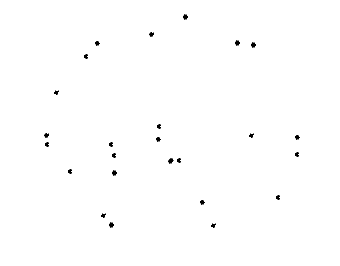compute convex hull of `points: Point3d[]` `const hull: Point3d[] = [];`
`const interior: Point3d[] = [];`
`Point3dArray.computeConvexHullXY(points, hull, interior, true);`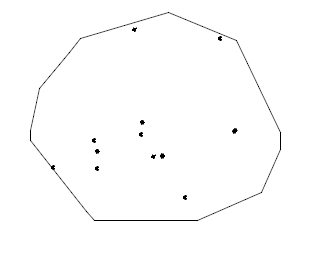One step `points: Point3d[]` to Polyface `const polyface = PolyfaceBuilder.pointsToTriangulatedPolyface(points);`
IndexedPolyface with all the points triangulated.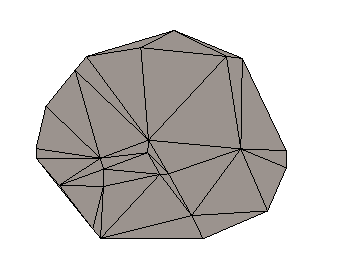Unit Test

• source: imodeljs\core\geometry\src\test\topology\InsertAndRetriangulateContext.test.ts
• test name: "TriangulateInHull"
• output: imodeljs\core\geometry\src\test\output\InsertAndRetriangulateContext\TriangulateInHull.imjs

## Triangulate points "between linestrings"

4 and 6 sided polygons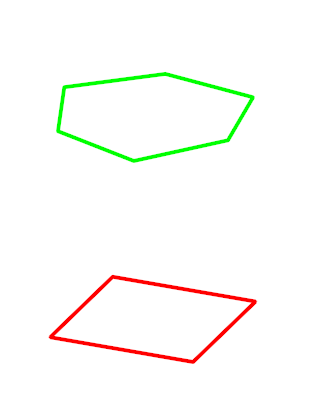Same polygons displayed with `<br>`handles to indicate the two linestrings have edge subdivision mismatch `<br>` in addition to the different cornering angles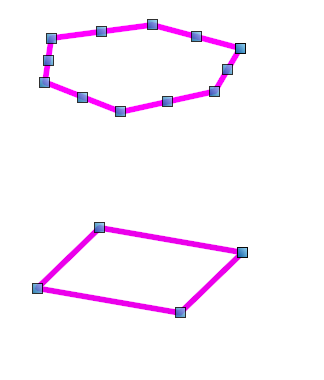triangles constructed "between" the polygons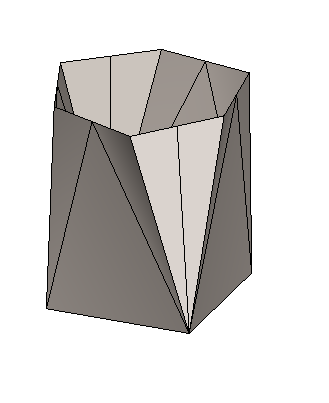Same polygons, another mix of points along edges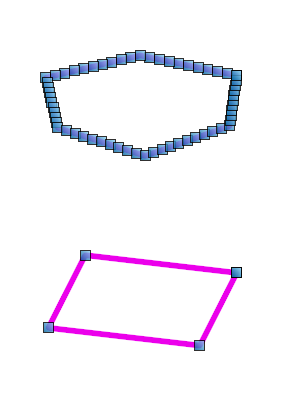triangles constructed "between" the polygons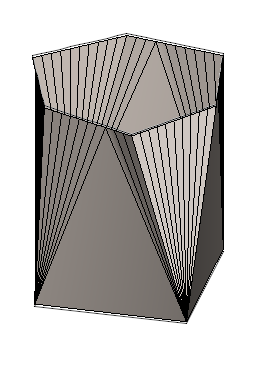Unit Test

• source: imodeljs\core\geometry\src\test\Polyface\GreedyTriangulationBetweenLineStrings.test.ts
• test set: `describe("GreedyTriangulationBetweenLineStrings"`
• test name: `quadStar`